Aug 30, 2023

# Query best practices: When should you use the IN instead of the OR operator?

Bohan ZhangThe original idea of a declarative query language like SQL is that you ask a database management system (DBMS) to give you the answer you want it to and not how to compute it. It is up to the DBMS's query optimizer (aka planner, compiler) to determine the most efficient execution plan for a SQL query. Ideally, the DBMS should choose the same optimal plan if you ask the same question using different SQL commands.

But this is not always the case, especially in beloved open-source DBMSs like PostgreSQL. Query performance often varies depending on how you write queries. We have seen some OtterTune customers where a simple change of SQL leads to a remarkable 10,000x speedup

To better understand this problem, in this article, we focus on queries utilizing the IN and OR operators to compute a disjunction in their WHERE clauses. Below are two example queries, both yielding identical results, yet their performance differs wildly:

``````SELECT * FROM item WHERE id IN (1, 2, 3)  --- IN operator
SELECT * FROM item WHERE id = 1 OR id = 2 OR id = 3   --- OR operator ``````

We will analyze the performance results obtained from our experiments in PostgreSQL, comparing IN operator and OR operator queries under various scenarios. By exploring the reasons behind these performance differences, our goal is to recommend best practices for writing efficient queries.

### Experiment setup

We use the following example table containing item information. Each row in the table includes the item name, price, quantity, and a unique ID serving as the primary key. We also create a composite index on (name, quantity).

Here is the DDL commands to create this table and the INSERT statement to populate it with 10m tuples of random data:

``````CREATE TABLE item (
id INT PRIMARY KEY,
name VARCHAR(32),
price INT,
quantity INT
);
CREATE INDEX idx_item_name_quantity ON item (name, quantity);

INSERT INTO item
SELECT id,
SUBSTR(MD5(RANDOM()::text), 0, 32) AS name,
(RANDOM() * 10000)::int AS price,
(RANDOM() * 10000)::int AS quantity
FROM generate_series(1, 10000000) AS t(id)

``````

We used a PostgreSQL v14 database on a db.t3.medium Amazon RDS instance equipped with 2 CPUs and 4GB RAM. The storage capacity is 200GB (gp2 storage). All experiments are conducted with PostgreSQL’s Just-in-Time Compilation (JIT) feature enabled to compile predicates into machine code using LLVM. We conduct three consecutive runs for each query and report the average latency.

We use OtterTune’s AI-powered service to optimize PostgreSQL’s configuration knobs (e.g., AWS’s ParameterGroup). We also pre-load the entire table into memory using pg_prewarm:

``````CREATE EXTENSION pg_prewarm;
SELECT pg_prewarm('item')

``````

### Single attribute filters

We first examine queries that reference a single attribute in their WHERE clause. These represent the majority of the queries that we see in OtterTune’s automated query tuning feature.

#### Single indexed attribute

We measure PostgreSQL’s performance on queries that retrieve multiple item records on an indexed attribute. We first use a single IN operator in the query’s WHERE clause and then run the same lookups using multiple OR clauses. We run multiple variations of each query with an increasing number of id lookups.

``````SELECT * FROM item WHERE id IN (?); –-  IN Clause
SELECT * FROM item WHERE id = ? OR id = ? OR ... ; -- OR Clauses``````

The chart below shows the performance results. When the number of predicates in the filter condition is small, the performance difference between the IN clause and OR clauses is negligible. However, as the number of predicates per query increases, PostgreSQL executes the IN clause query much faster than the query with the OR clauses. Again, both queries are computing the same answer on the same table so the run times should be the same. But the performance gap between the two queries expands with a larger number of predicates.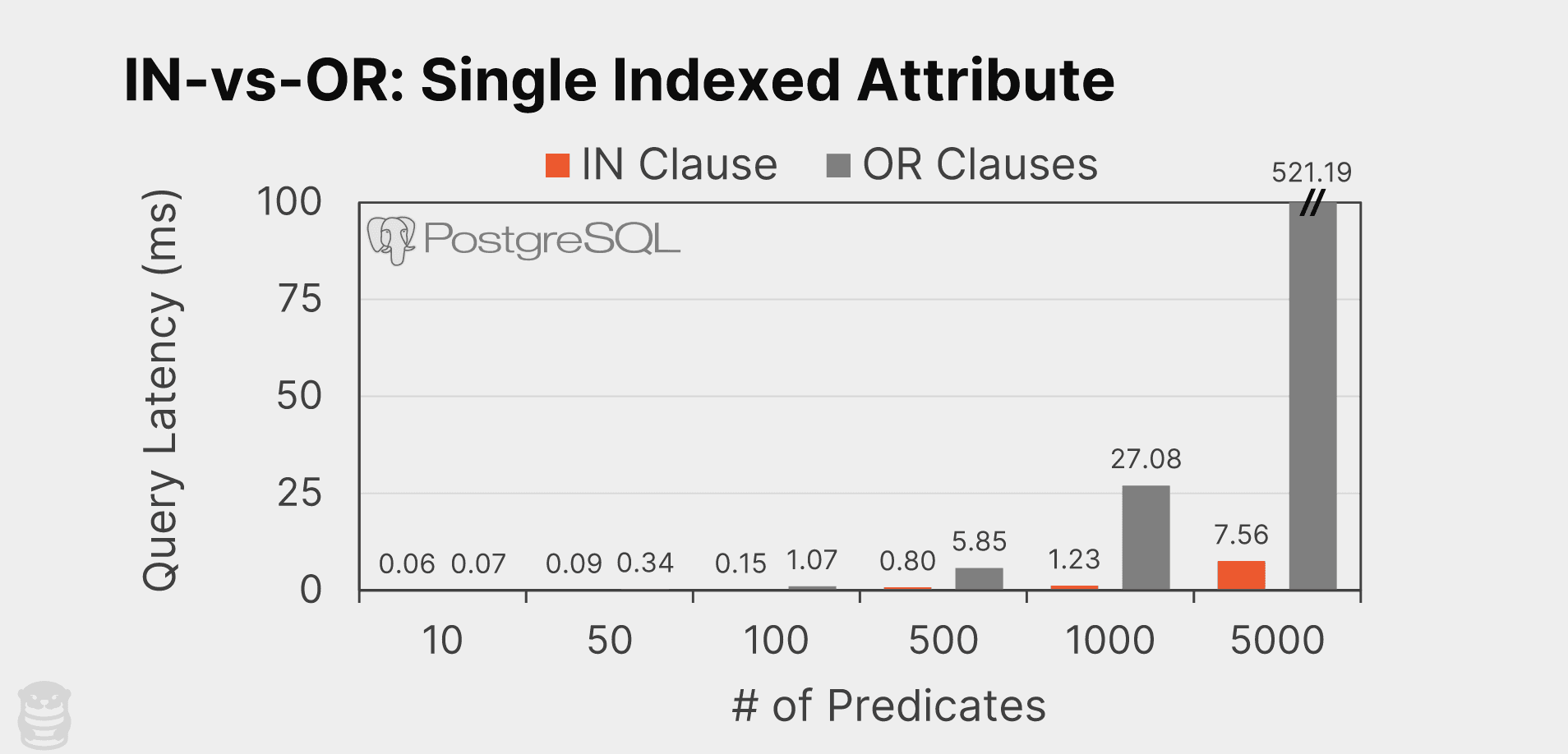At 5000 predicates, the IN clause query is approximately 69x faster than the OR clause (7.56ms vs. 521.19ms). To understand why this is the case, we examine the query plans for both the IN clause and OR clause using PostgreSQL’s EXPLAIN feature. For brevity, we consider the queries when using only three predicates:

``````ottertune=> EXPLAIN SELECT * FROM item WHERE id IN (1,2,3);
QUERY PLAN
-----------------------------------------------------------------------------------
Index Scan using item_pkey on item  (cost=0.43..17.36 rows=3 width=44)
Index Cond: (id = ANY ('{1,2,3}'::integer[]))``````
``````ottertune=> EXPLAIN SELECT * FROM item WHERE id = 1 OR id = 2 OR id = 3;
QUERY PLAN
-----------------------------------------------------------------------------------
Bitmap Heap Scan on item  (cost=12.90..24.52 rows=3 width=70)
Recheck Cond: ((id = 1) OR (id = 2) OR (id = 3))
Heap Blocks: exact=1
->  BitmapOr  (cost=12.90..12.90 rows=3 width=0)
->  Bitmap Index Scan on item_pkey  (cost=0.00..4.30 rows=1 width=0)
Index Cond: (id = 1)
->  Bitmap Index Scan on item_pkey  (cost=0.00..4.30 rows=1 width=0)
Index Cond: (id = 2)
->  Bitmap Index Scan on item_pkey  (cost=0.00..4.30 rows=1 width=0)
Index Cond: (id = 3)``````

The IN clause query uses PostgreSQL’s Index Scan access method to retrieve data via an index. The DBMS scans the primary key index (item_pkey) to find which rows satisfy the query condition (i.e., id is any value in {1,2,3}) and then retrieves the matching rows from the table.

The OR clause query utilizes PostgreSQL’s Bitmap Index Scan access method to scan the index and construct a bitmap to track matching rows. Each entry in the bitmap represents a row position in the table and indicates whether that row satisfies the query conditions as the DBMS scans the table’s primary key index (item_pkey).

After populating the bitmap, the DBMS retrieves the table pages containing matching records. The DBMS creates three bitmaps for each predicate in the disjunction clause. That is, PostgreSQL creates a bitmap to track (id = 1), another bitmap for (id = 2), and a third one for (id = 3). It then performs three index scans to populate each bitmap. But maintaining separate bitmaps is unnecessary because id is the primary key, which means that each predicate will match one and only one tuple. PostgreSQL then combines these bitmaps using a bitwise OR operation.

The number of bitmaps that PostgreSQL uses for these OR clause queries increases proportionally with the number of predicates in the WHERE clause. For example, when there are 5000 OR operations, the DBMS creates 5000 bitmaps, which causes a considerable slowdown compared to the IN clause that only requires one index scan. As the number of conditions increases, the DBMS OR clause incurs larger overhead in bitmap creation and combination, resulting in a notable performance gap compared to the IN clause.

### IN vs. OR: single unindexed attribute

We next examined queries that filtered based on a single unindexed attribute (price). We again do the same comparison where we generate two variations of the same query: one using a single IN clause and one using multiple OR clauses with equality predicates. We then scale up the number of comparisons per query.

``````SELECT * FROM item WHERE price IN (?); –-  IN Clause
SELECT * FROM item WHERE price = ? OR price = ? OR price = ? OR ... ; -- OR Clauses``````

As illustrated below, once again the IN clause queries outperforms the multiple OR clauses variants. The difference is much larger than the queries on the indexed attribute above and the gap widens even more with an increase in the number of predicates. With 5000 predicates, PostgreSQL executes the IN clause query approximately 288x faster than the OR clause (1.8 seconds vs. 518.2 seconds).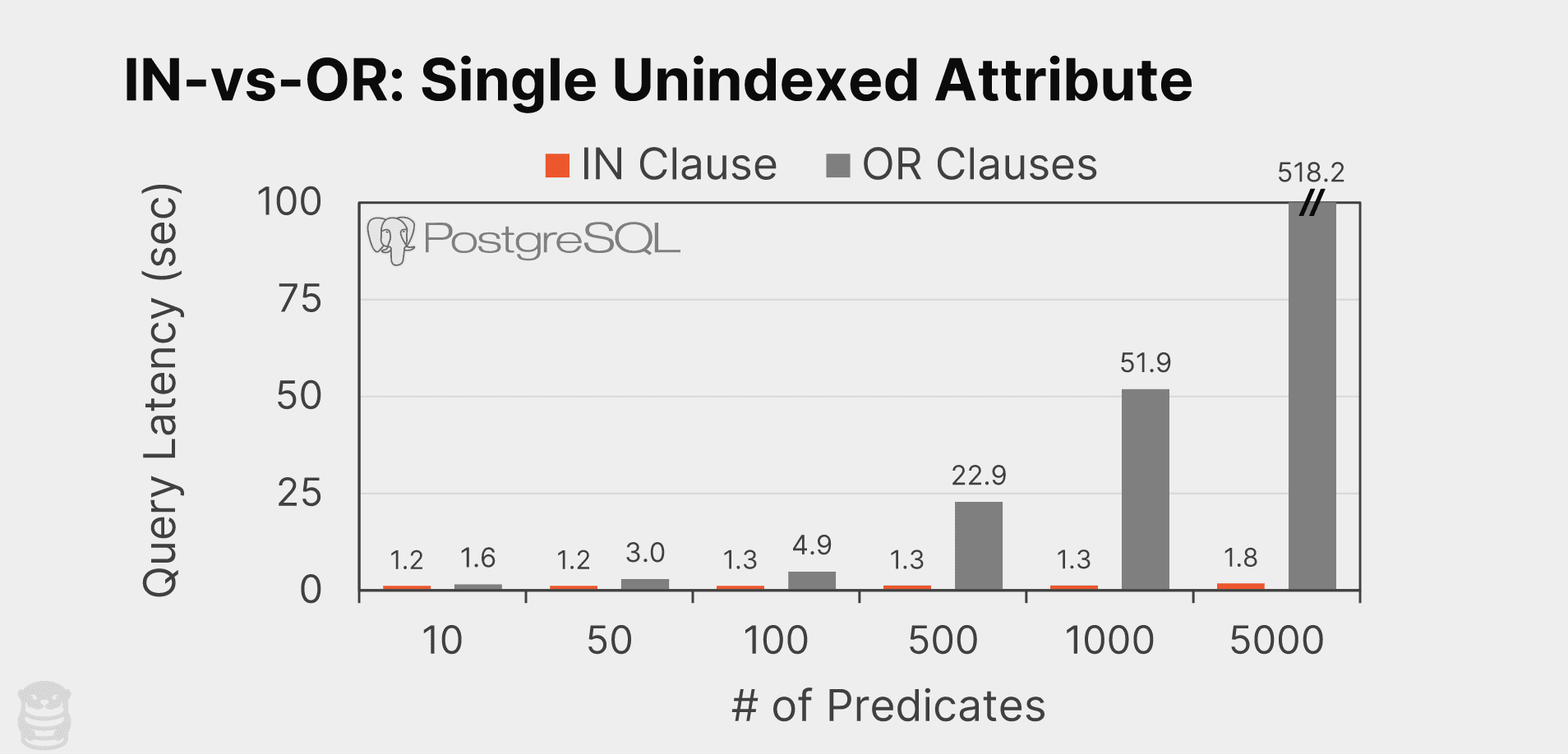Let's also compare two query plans to observe their differences:

``````ottertune=> EXPLAIN SELECT * FROM item WHERE price IN (1,2,3);
QUERY PLAN
-----------------------------------------------------------------------------------
Gather  (cost=1000.00..152048.39 rows=2988 width=44)
Workers Planned: 2
->  Parallel Seq Scan on item  (cost=0.00..150749.59 rows=1245 width=44)
Filter: (price = ANY ('{1,2,3}'::integer[]))``````
``````ottertune=> EXPLAIN SELECT * FROM item WHERE price = 1 OR price = 2 OR price = 3;
QUERY PLAN
-----------------------------------------------------------------------------------
Gather  (cost=1000.00..167673.27 rows=2987 width=44)
Workers Planned: 2
->  Parallel Seq Scan on item  (cost=0.00..166374.57 rows=1245 width=44)
Filter: ((price = 1) OR (price = 2) OR (price = 3))``````

Unlike in the previous experiment where the two query plans are different (Index Scan vs. Bitmap Index Scan), this time the plans are the same. As shown above, both queries execute Sequential Scans that read every page in the table and evaluate whether the price satisfies the filtering conditions on a row-by-row basis. However, the manner in which PostgreSQL performs the value comparison for filtering differs between the two plans, which explains the large performance advantage of IN versus OR.

For the OR clause query, the DBMS sequentially compares each condition's value one by one. In the given example, it performs three comparisons for each row to determine if (price = 1), (price = 2), and then (price = 3). This evaluation approach means that for N predicates, the complexity of the filtering operation per row is O(N).

On the other hand, with the IN clause, PostgreSQL builds a temporary hash table populated with the elements in the query’s IN clause (ExecEvalHashedScalarArrayOp). Then as the DBMS scans each tuple, it probes this hash table to see whether the tuple's attribute matches with any entry. This hash-based evaluation has a more efficient complexity of O(1) since the DBMS only needs to perform one lookup in the hash table per tuple.

Hence, as the number of predicates increases in a query, the OR clause incurs significant overhead because the DBMS evaluates predicates for each row individually, in contrast to the more efficient IN clause.

### Multiple attribute filters

We also ran tests using queries that filter records on using equality predicates on two attributes. We first evaluate the scenarios when both attributes are either indexed or unindexed. We then examined queries where there is an index for only one of the attributes.

The spoiler for this part is that PostgreSQL generates the same plan for the IN query as it does for the OR query in each scenario. This means that their performance is the same. Thus, it does not matter whether you use the IN clause or the OR clause when querying on multiple attributes since they both generate the same query plan.

#### Two indexed attributes

The queries to filter the table on two attributes (name, quantity) using the the composite index (idx_item_name_quantity) are as follows:

``````SELECT * FROM item WHERE (name, quantity) IN (('a', 1), ('b', 2));
SELECT * FROM item WHERE (name='a' AND quantity=1) OR (name='b' AND quantity=2)

``````

The query plan is as follows:

``````Bitmap Heap Scan on item  (cost=9.14..13.16 rows=1 width=44)
Recheck Cond: ((((name)::text = 'a'::text) AND (quantity = 1)) OR (((name)::text = 'b'::text) AND (quantity = 2)))
->  BitmapOr  (cost=9.14..9.14 rows=1 width=0)
->  Bitmap Index Scan on idx_item_name_quantity  (cost=0.00..4.57 rows=1 width=0)
Index Cond: (((name)::text = 'a'::text) AND (quantity = 1))
->  Bitmap Index Scan on idx_item_name_quantity  (cost=0.00..4.57 rows=1 width=0)
Index Cond: (((name)::text = 'b'::text) AND (quantity = 2))``````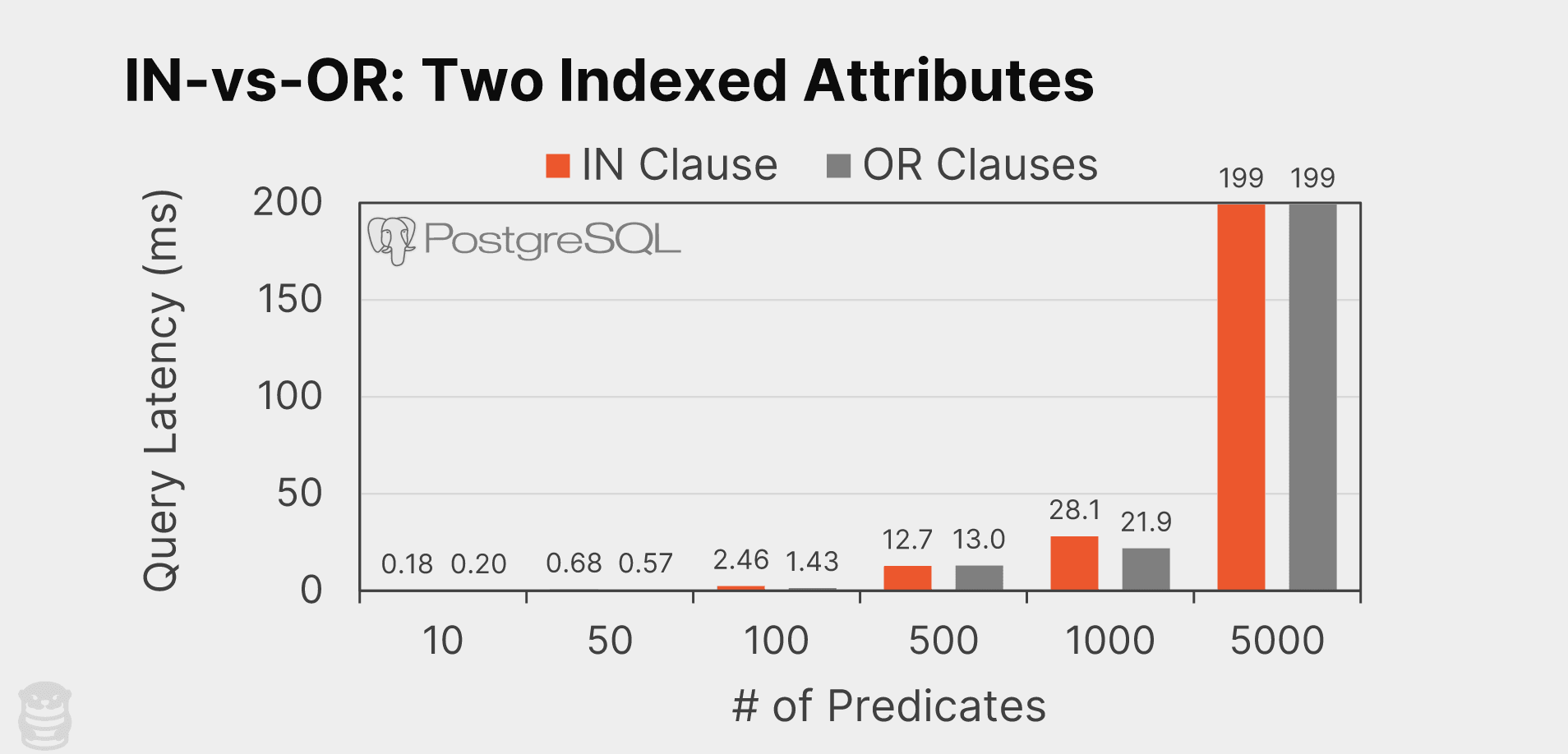These results show that the execution time is about the same for both queries. In both scenarios, PostgreSQL executes a separate Bitmap Index Scan per predicate in the WHERE clause as it did with the OR clause query with a single indexed attribute. That is, the DBMS does not apply any optimizations for the IN clause query.

#### Two unindexed attributes

We next consider queries that filter on two unindexed attributes (price, quantity):

``````SELECT * FROM item WHERE (price, quantity) IN ((1, 1), (2, 2));
SELECT * FROM item WHERE (price=1 AND quantity=1) OR (price=2 AND quantity=2)

``````

Once again we see that PostgreSQL generates the same plans for both queries:

``````Gather  (cost=1000.00..177791.33 rows=1 width=44)
Workers Planned: 2
->  Parallel Seq Scan on item  (cost=0.00..176791.23 rows=1 width=44)
Filter: (((price = 1) AND (quantity = 1)) OR ((price = 2) AND (quantity = 2)))``````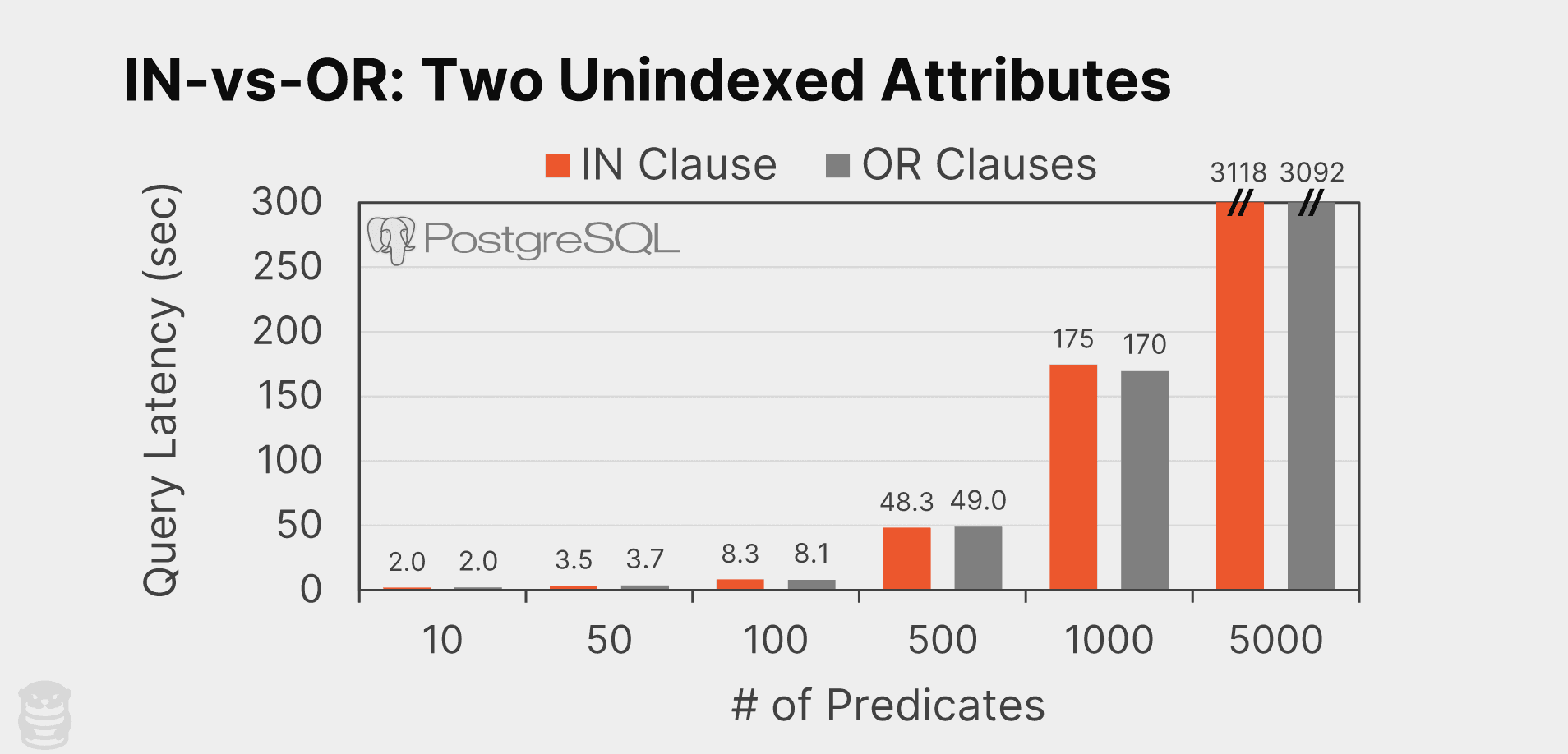The above results show that the performance of the two queries are again essentially the same. This is because PostgreSQL does not build a temporary hash table to speed up IN clause evaluation when there is more than one attribute. PostgreSQL notably also does not support multi-attribute hash indexes either (see this psql-hackers email thread discussing this limitation way back in 1998!).

#### One indexed + one unindexed

Lastly, we test queries where there is only an index for one of the attributes (id) and one unindexed attribute (price):

``````SELECT * FROM item WHERE (id, price) IN ((1, 1), (2, 2));
SELECT * FROM item WHERE (id=1 AND price=1) OR (id=2 AND price=2)

``````

And like the other tests, PostgreSQL chooses the same plan for both queries:

`````` Bitmap Heap Scan on item  (cost=8.89..16.90 rows=1 width=36)
Recheck Cond: ((id = 1) OR (id = 2))
Filter: (((id = 1) AND (price = 1)) OR ((id = 2) AND (price = 2)))
->  BitmapOr  (cost=8.89..8.89 rows=2 width=0)
-> Bitmap Index Scan on item_pkey (cost=0.00..4.44 rows=1 width=0)
Index Cond: (id = 1)
-> Bitmap Index Scan on item_pkey (cost=0.00..4.44 rows=1 width=0)
Index Cond: (id = 2)``````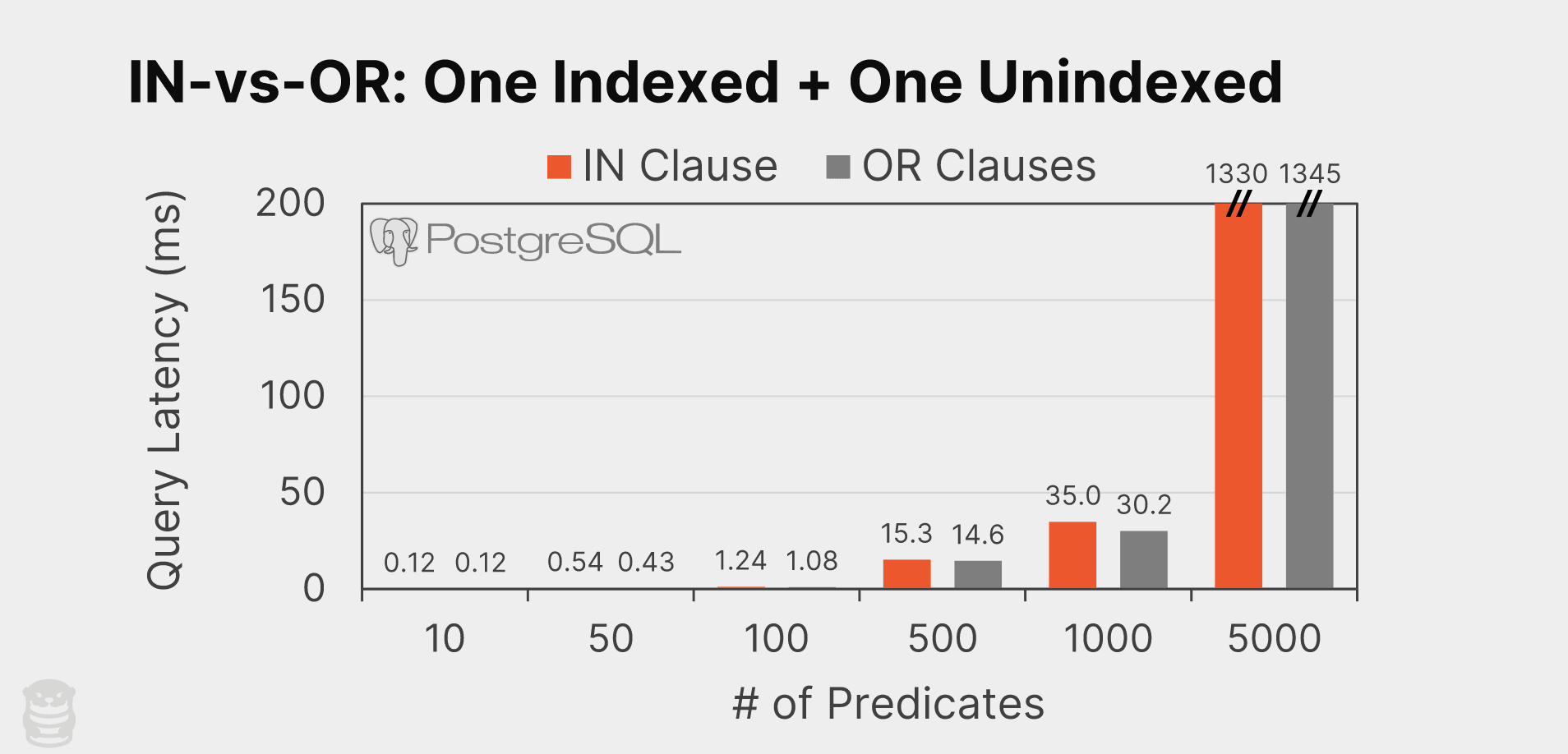And again we see that the queries execution times are unsurprisingly the same.

### Best practices

Based on our results, we find that PostgreSQL consistently exhibits equivalent or better performance for queries with an IN clause than OR clauses when filtering on a single attribute. This is especially true for queries with a large number of predicates. For small condition sizes (e.g., less than 10), the performance difference between the two query types is negligible.

When filtering on multiple attributes, both the IN and OR clauses have the same performance because PostgreSQL chooses the same plan for both queries.

Thus, we advise that you should always use IN clauses with PostgreSQL, making it a preferred choice for better efficiency.

Try OtterTune for free. Start using AI to optimize your PostgreSQL or MySQL databases running on Amazon RDS or Aurora.

Try OtterTune for free. Start using AI to optimize your PostgreSQL or MySQL databases running on Amazon RDS or Aurora.

Get Started